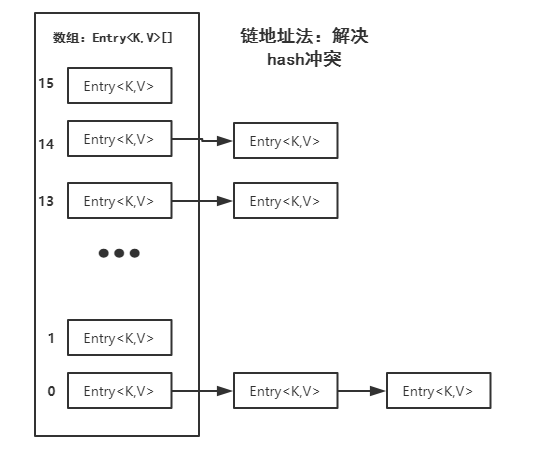# 【java基础】HashMap实现原理及源码分析

hash算法冲突解决：

开放定址法：

## 2.HashMap实现原理

1. HashMap底层实现的数据结构是什么？
2. HashMap中的hash()函数的作用？
3. HashMap可以存储null的键吗？
4. HashMap是什么时候做Bucket（桶）的初始化的？
5. HashMap是使用那种方式处理冲突的？
6. HashMap默认的存储大小，默认负载因子是多少？

ok,现在让我们进入HashMap的源码，去一一解惑！

## 3.首先看一下HashMap的底层数据结构：数组+链表/**
* 默认初始容量，默认为2的4次方 = 16，2的n次方是为了加快hash计算速度，；；减少hash冲突，，，h & (length-1)，，1111111
*/
static final int DEFAULT_INITIAL_CAPACITY = 1 << 4; // aka 16

/**
* 最大容量，默认为2的30次方，
*/
static final int MAXIMUM_CAPACITY = 1 << 30;

/**
* 默认负载因子，默认为0.75
*/
static final float DEFAULT_LOAD_FACTOR = 0.75f;

/**
*当数组表还没扩容的时候，一个共享的空表对象
*/
static final Entry<?,?>[] EMPTY_TABLE = {};

/**
* 数组表，大小可以改变，且大小必须为2的幂
*/
transient Entry<K,V>[] table = (Entry<K,V>[]) EMPTY_TABLE;

/**
* 当前Map中key-value映射的个数
*/
transient int size;

/**
* 下次扩容阈值，当size > capacity * load factor时，开始扩容
*/
int threshold;

/**
* 负载因子
*/
final float loadFactor;

/**
* Hash表结构性修改次数，用于实现迭代器快速失败行为
*/
transient int modCount;

static class Entry<K,V> implements Map.Entry<K,V> {
final K key;
V value;
Entry<K,V> next;//指向下一个Entry
int hash;

/**
* Creates new entry.
*/
Entry(int h, K k, V v, Entry<K,V> n) {
value = v;
next = n;
key = k;
hash = h;
}

public final K getKey() {
return key;
}

public final V getValue() {
return value;
}

public final V setValue(V newValue) {
V oldValue = value;
value = newValue;
return oldValue;
}

public final boolean equals(Object o) {
if (!(o instanceof Map.Entry))
return false;
Map.Entry e = (Map.Entry)o;
Object k1 = getKey();
Object k2 = e.getKey();
if (k1 == k2 || (k1 != null && k1.equals(k2))) {
Object v1 = getValue();
Object v2 = e.getValue();
if (v1 == v2 || (v1 != null && v1.equals(v2)))
return true;
}
return false;
}

public final int hashCode() {
return Objects.hashCode(getKey()) ^ Objects.hashCode(getValue());
}

public final String toString() {
return getKey() + "=" + getValue();
}

/**
* This method is invoked whenever the value in an entry is
* overwritten by an invocation of put(k,v) for a key k that's already
* in the HashMap.
*/
void recordAccess(HashMap<K,V> m) {
}

/**
* This method is invoked whenever the entry is
* removed from the table.
*/
void recordRemoval(HashMap<K,V> m) {
}
}

Entry是一个键值对类，用来保存键值对，而其内部维护了一个next变量用来指向下一个Entry键值对。如上图所示。

## HashMap的构造函数：

    /**
* 传入初始化容量，负载因子来构造HashMap
*/
public HashMap(int initialCapacity, float loadFactor) {
//容量小于0，抛出异常
if (initialCapacity < 0)
throw new IllegalArgumentException("Illegal initial capacity: " +
initialCapacity);
//容量大于HashMap最大容量2^30，初始化容量就设为最大容量
if (initialCapacity > MAXIMUM_CAPACITY)
initialCapacity = MAXIMUM_CAPACITY;
//如果负载因子<=0或者负载因子不是数值，NaN是一个计算机数值，表示不是数值，比如0/0结果就
//是NaN
if (loadFactor <= 0 || Float.isNaN(loadFactor))
throw new IllegalArgumentException("Illegal load factor: " +
loadFactor);

this.loadFactor = loadFactor;

//扩容阀值设置为初始容量
threshold = initialCapacity;
init();
}

/**
* 使用默认负载因子0.75来实例化
*/
public HashMap(int initialCapacity) {
this(initialCapacity, DEFAULT_LOAD_FACTOR);
}

/**
* 使用默认初始容量和默认负载因子实例化
*/
public HashMap() {
this(DEFAULT_INITIAL_CAPACITY, DEFAULT_LOAD_FACTOR);
}

## put函数代码如下：

/**
* 将键值对存储到HashMap
*/
public V put(K key, V value) {
//如果表是空表，即第一次调用put()函数时，执行inflateTable()函数，初始化table
if (table == EMPTY_TABLE) {
inflateTable(threshold);
}
//如果键值null，就执行putForNullKey()函数，可以看出HashMap是可以存储key为Null的键值对
//事实上HashMap把key为null的键值对放在table这个位置，后面可证。
if (key == null)
return putForNullKey(value);
//这里调用hash(key)函数，将key传入，获取该键对应的hash值
int hash = hash(key);
//根据上面得到的hash值来得到存储在table数组中的索引位置。
int i = indexFor(hash, table.length);
//如果table在i这个索引位置已经有Entry对象了，就查找该链表上是否已存在该key.如果存在就用
//新的value来覆盖旧的value,并返回旧的value
for (Entry<K,V> e = table[i]; e != null; e = e.next) {
Object k;
if (e.hash == hash && ((k = e.key) == key || key.equals(k))) {
V oldValue = e.value;
e.value = value;
e.recordAccess(this);
return oldValue;
}
}

modCount++;
//该索引位置不存在该key,则将该键值对插入到i索引位置（头插入）。
addEntry(hash, key, value, i);
return null;
}

## inflateTable(int toSize)函数代码如下：

    /**
* 扩容table表
*/
private void inflateTable(int toSize) {
// 根据toSize返回一个2的幂次方数值。后面会讲为啥必须是2的幂次方！
int capacity = roundUpToPowerOf2(toSize);
//设置扩容阀值
threshold = (int) Math.min(capacity * loadFactor, MAXIMUM_CAPACITY + 1);
//这里才对table进行初始化构造，初始容量为capacity
table = new Entry[capacity];
initHashSeedAsNeeded(capacity);
}

## int hash(Object k)函数代码如下：

//hash函数对k的哈希码进行了多次的右移和异或操作，目的是让哈希值更加均匀的分散在Bucket上
final int hash(Object k) {
int h = hashSeed;
if (0 != h && k instanceof String) {
return sun.misc.Hashing.stringHash32((String) k);
}
h ^= k.hashCode();

// This function ensures that hashCodes that differ only by
// constant multiples at each bit position have a bounded
// number of collisions (approximately 8 at default load factor).
h ^= (h >>> 20) ^ (h >>> 12);
return h ^ (h >>> 7) ^ (h >>> 4);
}

## static int indexFor(int h, int length)函数代码如下：

    /**
* 使用取余法将hash值散列到各个Bucket上。&运算比取余操作性能更高。
*/
static int indexFor(int h, int length) {
return h & (length-1);
}

    10100101 11000100 00100101
&   00000000 00000000 00001111
----------------------------------
00000000 00000000 00000101    //高位全部归零，只保留末四位void addEntry(int hash, K key, V value, int bucketIndex) {
//如果键值对数量大于扩容阀值且在bucketIndex这个位置已经有Entry对象了，就扩大HashMap的储存
//容量，这里可以看出HashMap选择在原来的存储容量扩大2倍。
if ((size >= threshold) && (null != table[bucketIndex])) {
resize(2 * table.length);
hash = (null != key) ? hash(key) : 0;
bucketIndex = indexFor(hash, table.length);
}

createEntry(hash, key, value, bucketIndex);
}

# 为何HashMap的数组长度一定是2的次幂？

void resize(int newCapacity) {
Entry[] oldTable = table;
int oldCapacity = oldTable.length;
if (oldCapacity == MAXIMUM_CAPACITY) {
threshold = Integer.MAX_VALUE;
return;
}

Entry[] newTable = new Entry[newCapacity];
transfer(newTable, initHashSeedAsNeeded(newCapacity));
table = newTable;
threshold = (int)Math.min(newCapacity * loadFactor, MAXIMUM_CAPACITY + 1);
}

void transfer(Entry[] newTable, boolean rehash) {
int newCapacity = newTable.length;
//for循环中的代码，逐个遍历链表，重新计算索引位置，将老数组数据复制到新数组中去（数组不存储实际数据，所以仅仅是拷贝引用而已）
for (Entry<K,V> e : table) {
while(null != e) {
Entry<K,V> next = e.next;
if (rehash) {
e.hash = null == e.key ? 0 : hash(e.key);
}
int i = indexFor(e.hash, newCapacity);
//将当前entry的next链指向新的索引位置,newTable[i]有可能为空，有可能也是个entry链，如果是entry链，直接在链表头部插入。
e.next = newTable[i];
newTable[i] = e;
e = next;
}
}
}

hashMap的数组长度一定保持2的次幂，比如16的二进制表示为 10000，那么length-1就是15，二进制为01111，同理扩容后的数组长度为32，二进制表示为100000，length-1为31，二进制表示为011111。从下图可以我们也能看到这样会保证低位全为1，而扩容后只有一位差异，也就是多出了最左位的1，这样在通过 h&(length-1)的时候，只要h对应的最左边的那一个差异位为0，就能保证得到的新的数组索引和老数组索引一致(大大减少了之前已经散列良好的老数组的数据位置重新调换)，个人理解。还有，数组长度保持2的次幂，length-1的低位都为1，会使得获得的数组索引index更加均匀，比如：我们看到，上面的&运算，高位是不会对结果产生影响的（hash函数采用各种位运算可能也是为了使得低位更加散列），我们只关注低位bit，如果低位全部为1，那么对于h低位部分来说，任何一位的变化都会对结果产生影响，也就是说，要得到index=21这个存储位置，h的低位只有这一种组合。这也是数组长度设计为必须为2的次幂的原因。如果不是2的次幂，也就是低位不是全为1此时，要使得index=21，h的低位部分不再具有唯一性了，哈希冲突的几率会变的更大，同时，index对应的这个bit位无论如何不会等于1了，而对应的那些数组位置也就被白白浪费了。

get方法

public V get(Object key) {
//如果key为null,则直接去table处去检索即可。
if (key == null)
return getForNullKey();
Entry<K,V> entry = getEntry(key);
return null == entry ? null : entry.getValue();
}

get方法通过key值返回对应value，如果key为null，直接去table处检索。我们再看一下getEntry这个方法

final Entry<K,V> getEntry(Object key) {

if (size == 0) {
return null;
}
//通过key的hashcode值计算hash值
int hash = (key == null) ? 0 : hash(key);
//indexFor (hash&length-1) 获取最终数组索引，然后遍历链表，通过equals方法比对找出对应记录
for (Entry<K,V> e = table[indexFor(hash, table.length)];
e != null;
e = e.next) {
Object k;
if (e.hash == hash &&
((k = e.key) == key || (key != null && key.equals(k))))
return e;
}
return null;
}

https://www.cnblogs.com/chenpi/p/5280304.html

https://www.cnblogs.com/chengxiao/p/6059914.html

https://www.cnblogs.com/zhengwang/p/8136164.html